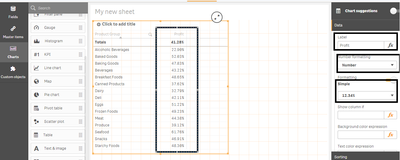Announcements
Sept. 27, 10AM ET Do more with Qlik, Insight Advisor – our intelligent AI-assistant in Qlik Sense: Register
cancel
Showing results for
Did you mean:Creator

## Creating Traffic lights in Qliksense Table

Creating Traffic lights in Qliksense Table-

I have some data of Sales and Product.

Step1- Select the Table from the chartsStep2- From the data select the Product Group as dimension and Expression like as to calculate Profit-

=(sum(Sales)-Sum(Cost))/Sum(Sales)

Step3- Change the properties of Measure as like below-

1. Lable- Profit
2. Number Formatting- Number
3. Formatting- Simple (12.34%)Step4-  Create another measure and insert the Unicode symbol for Black circle i.e. Chr(11044).

1. Create label as Traffic
2. In Text color expression put the below mentioned expression-

=if(((Sum(Sales)-Sum(Cost))/Sum(Sales))>0.6,lightgreen(),

if(((Sum(Sales)-Sum(Cost))/Sum(Sales))>0.4 and ((Sum(Sales)-Sum(Cost))/Sum(Sales))<0.6, Yellow(),

if(((Sum(Sales)-Sum(Cost))/Sum(Sales))<0.4, lightRed()

)

)

)

You will get result as-Sumit Kumar Srivastava
1 Solution

Accepted SolutionsCreator
Author

This is created by expression as conditional, so it is not possible to select direct from table. You have to create some flag for this and take that flag in filter pane to filter the traffic light.

Sumit Kumar Srivastava
2 RepliesContributor III

How can we filter the traffic lights in the table?Creator
Author

This is created by expression as conditional, so it is not possible to select direct from table. You have to create some flag for this and take that flag in filter pane to filter the traffic light.

Sumit Kumar SrivastavaTags
Community Browser